# RS Aggarwal Solutions Class 10 Chapter 9 Mean, Median, Mode of Grouped Data Ex 9d

## RS Aggarwal Solutions Class 10 Chapter 9 Mean, Median, Mode of Grouped Data,Cumulative Frequency Graph and O give Ex 9d

These Solutions are part of RS Aggarwal Solutions Class 10. Here we have given RS Aggarwal Solutions Class 10 Chapter 9 Mean, Median, Mode of Grouped Data,Cumulative Frequency Graph and O give Ex 9d.

Other Exercises

Question 1.
Solution:
Let assumed mean be 35, h = 10, now we have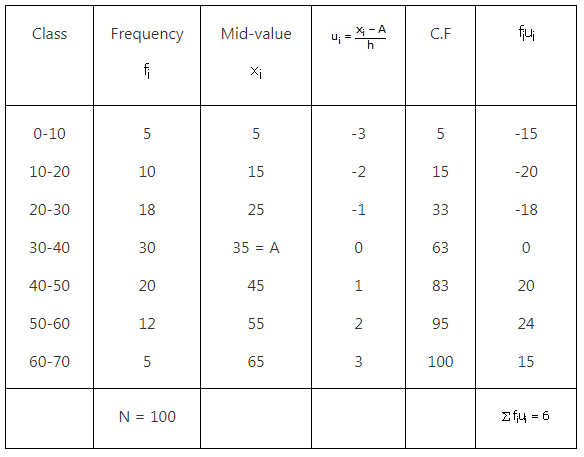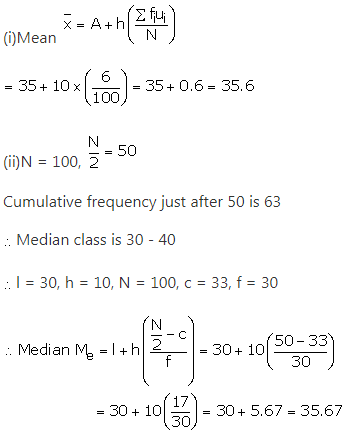(iii) Mode = 3 × median – 2 × mean
= 3 × 35.67 – 2 × 35.6 = 107.01 – 71.2
= 35.81
Thus, Mean = 35.6, Median = 35.67 and Mode = 35.81.

Question 2.
Solution:Question 3.
Solution:Question 4.
Solution:Question 5.
Solution:
Let the assumed mean A be 145.Class interval h = 10.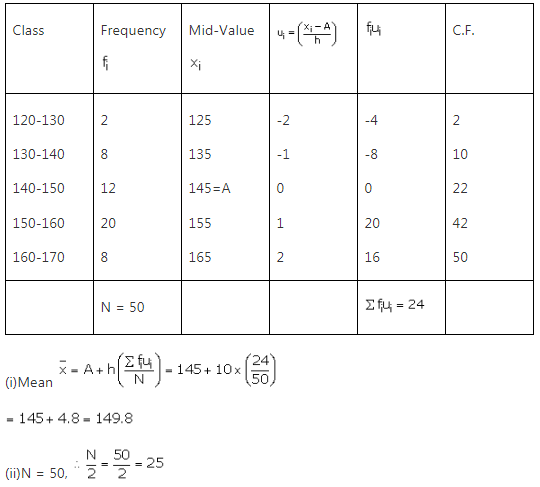Cumulative frequency just after 25 is 42
Corresponding median class is 150 – 160
Cumulative frequency before median class, c = 22
Median class frequency f = 20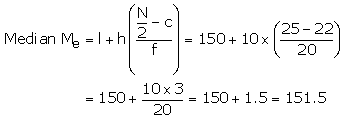(iii) Mode = 3 median – 2 mean
= 3 151.5 – 2 149.8 = 454.5 – 299.6
= 154.9
Thus, Mean = 149.8, Median = 151.5, Mode = 154.9.

Question 6.
Solution: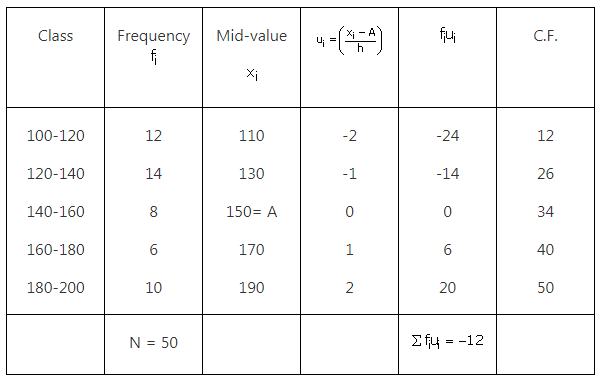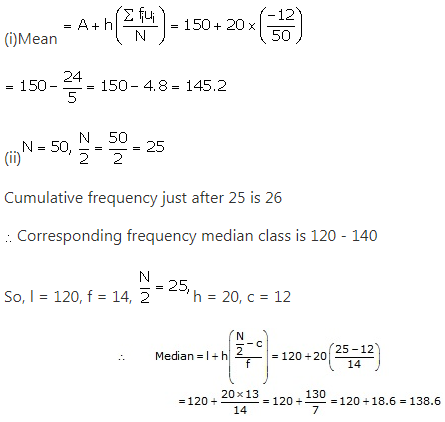(iii) Mode = 3 Median – 2 Mode
= 3 138.6 – 2 145.2
= 415.8 – 190.4
= 125.4
Hence, Mean = 145.2, Median = 138.6 and Mode = 125.4.

Question 7.
Solution: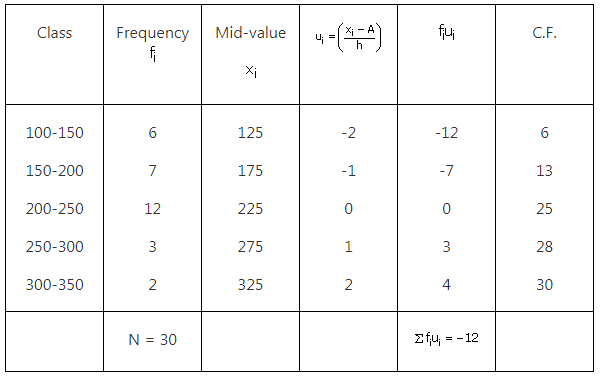Let assumed mean = 225 and h = 50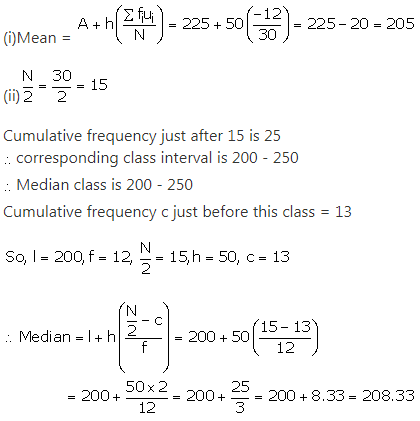Hence, Mean = 205 and Median = 208.33.

We hope the RS Aggarwal Solutions Class 10 Chapter 9 Mean, Median, Mode of Grouped Data Ex 9d help you. If you have any query regarding RS Aggarwal Solutions Class 10 Chapter 9 Mean, Median, Mode of Grouped Data Ex 9d, drop a comment below and we will get back to you at the earliest.